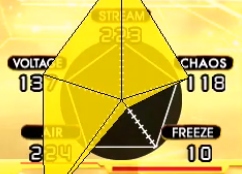This is a note provided by Aaron Tokunaga of Aaron In Japan about how the values of DDR’s “Groove Radar” are calculated.

Aaron has been involved in the DDR community since the very beginning and has acted as a bridge between the Eastern and Western communities. He has a book titled “Counting Combos” detailing the history of the Japanese DDR community and he is currently a contractor at KONAMI.

## Stream

Number of notes per minute = Floor(60 * Number of notes / Length of song[seconds])

If the number of notes oer minutes is:
over 300:Stream = ( Notes per minute – 139 ) * 100 / 161
under 300:Stream = Notes per minute / 3

Key values:
If the notes per minute value is 300, Stream = 100
If the notes per minute value is 461, Stream = 200

## Voltage

Average BPM = 60 * Song length[beats] / Song length[seconds]
Maximum note density = Highest number of notes in 4 beats (Including Shock Arrows)

Using these two formulas:
Maximum number of notes per minute = Floor( Average BPM * Maximum note density / 4 )

If the maximum number of notes per minutes is over 600, Voltage = ( Maximum number of notes per minute + 330 ) * 10 / 93
If the maximum number of notes per minutes is under 600, Voltage = Maximum number of notes per minute / 6

Key values:
Maximum number of notes per minutes = 600 : Voltage = 100、
Maximum number of notes per minutes = 1530 : Voltage = 200

* On DDR X3, in London EVOLVED Ver.A(Expert), the Maximum number of notes per minute max value was changed (1329→1530)

## Air

Number of jumps per minute = Floor( 60 * ( Number of simultaneously pressed notes + Number of shock arrows ) / Length of song[seconds] )

If the number of jumps per minute
Is over 55 : Air = ( Number of jumps per minute + 5 ) * 5 / 3
Is under 55 : Air = Number of jumps per minute * 20 / 11

Key values:
Number of jumps per minute = 55 : Air = 100
Number of jumps per minute = 115 : Air = 200

On DDR X3, TWINKLE♡HEART(Challenge) had the number of jumps per minute value changed (109→115)

## Freeze

Total Freeze Length = Total length of all freeze arrows[in beats]
* However, if there are two freeze arrows at the same time, ignore the shorter length (If they both end at the same time, ignore one of them)

Freeze Arrow rate = Floor( 10000 * Total Freeze Length / Length of song[beats] )

If the Freeze Arrow rate:
Is over 3500 : Freeze = ( Freeze Arrow rate + 2484 ) * 100 / 5984
Is under 3500 : Freeze = Freeze Arrow rate / 35

Key values:
Freeze arrow rate = 35.00% (3500) : Freeze = 100
Freeze arrow rate = 94.84% (9484) : Freeze = 200

## Chaos

Abnormality value = Abnormality value = Number of arrows * NOTE Color correction / Distance to previous note (including shock arrows)[beats]

* Number of arrows ： Single arrows are 1, Jumps/Double arrows are 2, Shock arrows depend on mode(Usually 4 on single, 8 on double)
* NOTE Color correction ： Based on the color of the arrow using the NOTE skin. RED=0, BLUE=2, YELLOW=4, GREEN=5
(Notes that are not color coded, such as the start of Freeze arrows or Shock arrows, are considered as if they were normal color coded steps using the NOTE skin)

Base Chaos Value = Total Abnormality value of all notes (including Shock arrows)

Total BPM change = Total change between the BPM and the stop
* When the BPM changes, it is the difference between the BPM before and after the change
* In the event of a stop, the BPM used is the one directly after the stop
* When the BPM change and stop are calculated at the same time (The BPM before and after the stop are different), only the values by the stop are considered

* Using POSSESSION as an example, The BPM changes are 370-185-0-370-185-0-185-370, so
Total BPM change = (370-185)+370+(370-185)+185+(370-185)=1110
BPM change per minute = 60 * Total BPM change / Length of song[seconds]

Chaos degree = Base Chaos Value * ( 1 + ( BPM change per minute / 1500 ) )
Chaos Unit Value = Chaos degree * 100 / Length of song[seconds]

If the Chaos Unit Value is :
Over 2000 : Chaos = ( Chaos Unit Value + 21605 ) * 100 / 23605
Under 2000 : Chaos = Chaos Unit Value / 20

* Note the Chaos value is tentative as there are results well over 2000. (CHAOS Challenge Single is calculated to have a Chaos Unit Value of 25605)」

Source